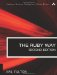# Section 16.8. Conclusion

### 16.7. Prettyprinting Objects

The purpose of the inspect method (and the p method that invokes it) is to show objects in human-readable form. In that sense, there is a connection with testing and debugging that justifies covering this here.

The only problem with p is that the object it prints out can be difficult to read. That is why we have the standard library pp, which adds a method of the same name.

Consider the following contrived object called my_obj:

`class MyClass   attr_accessor :alpha, :beta, :gamma   def initialize(a,b,c)     @alpha, @beta, @gamma = a, b, c   end end x = MyClass.new(2, 3, 4) y = MyClass.new(5, 6, 7) z = MyClass.new(7, 8, 9) my_obj = { x => y, z => [:p, :q] } p my_obj`

When we do the p, we get this output:

`{#<MyClass:0xb7eed86c @beta=3, @alpha=2,  @gamma=4>=>#<MyClass:0xb7eed72c @beta=6, @alpha=5, @gamma=7>,  #<MyClass:0xb7eed704 @beta=8, @alpha=7, @gamma=9>=>[:p, :q]}`

It's accurate, and it's technically readable. But it isn't pretty. Now let's require the pp library and use pp instead:

`require 'pp' # ... pp my_obj`

In this case, we get the following output:

`{#<MyClass:0xb7f7a050 @alpha=7, @beta=8, @gamma=9>=>[:p, :q],  #<MyClass:0xb7f7a1b8 @alpha=2, @beta=3, @gamma=4>=>   #<MyClass:0xb7f7a078 @alpha=5, @beta=6, @gamma=7>}`

At least it adds some spacing and line breaks. It's an improvement. But we can do better. Suppose we add the special pretty_print method to MyClass:

`class MyClass   def pretty_print(printer)     printer.text "MyClass(#@alpha, #@beta, #@gamma)"   end end`

The printer argument is passed in by the caller (or ultimately by pp). It is a text accumulator of class PP; we call its text method and give it a textual representation of self. The result we get follows:

`{MyClass(7, 8, 9)=>[:p, :q], MyClass(2, 3, 4)=>MyClass(5, 6, 7)}`

Of course, we can customize this as much as we want. We could put the instance variables on separate lines and indent them, for instance.

In fact, the pp library does have a number of facilities for "pp-enabling" your own classes. Methods such as object_group, seplist, breakable, and others are available for controlling comma separation, line breaks, and similar formatting tasks. For more details, consult the documentation on http://ruby-doc.org.The Ruby Way, Second Edition: Solutions and Techniques in Ruby Programming (2nd Edition)
ISBN: 0672328844
EAN: 2147483647
Year: 2004
Pages: 269
Authors: Hal Fulton

Similar book on Amazon